## The Boussinesq Approximation

### What Is the Boussinesq Approximation?

The Boussinesq approximation is a way to solve nonisothermal flow, such as natural convection problems, without having to solve for the full compressible formulation of the Navier-Stokes equations.

The Boussinesq approximation was a popular method for solving nonisothermal flow, particularly in previous years, as computational costs were lower when solving this method and convergence was more likely to be achieved. The approximation is accurate when density variations are small as this reduces the nonlinearity of the problem. It assumes that variations in density have no effect on the flow field, except that they give rise to buoyancy forces. In more practical terms, this approximation is typically used to model liquids around room temperature, natural ventilation in buildings, or dense gas dispersion in industrial set-ups.

While the Boussinesq approximation has been used to simplify the implementation of some CFD solvers, its use these days is becoming less prevalent. This is because it only slightly reduces the nonlinearity of the system and, with today's solvers and computational hardware, consequently leads to marginal reduction in computational costs. A larger computational cost difference between the full Navier-Stokes equations and the Boussinesq approximation may indicate that the Boussinesq approximation is not valid.

### Definition of the Boussinesq Approximation

The Navier-Stokes equations govern the motion of fluids. In the general case of a compressible fluid, this yields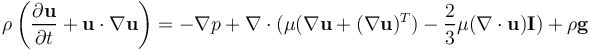where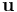is the fluid velocity,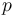is the fluid pressure,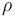is the fluid density,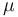is the fluid dynamic viscosity,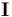is the identity matrix, and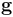is the acceleration due to gravity.

The Navier-Stokes equations are solved together with the continuity equation: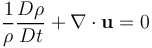The Boussinesq approximation states that the density variation is only important in the buoyancy term,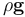, and can be neglected in the rest of the equation. This yields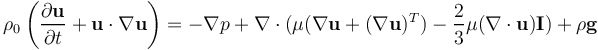where the temperature and pressure-dependent density,, have been replaced by a constant density,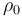, except in the body force term representing the buoyancy force.

Under the Boussinesq approximation, the continuity equationreduces to the incompressible form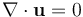, because the magnitude of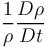is small with respect to the velocity gradients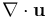. It follows that the term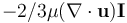in the Navier-Stokes equations is also equal to zero. It is also generally assumed that the viscosity,, is constant. The diffusion term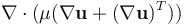can thus be rewritten as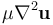to yield: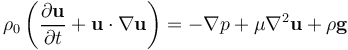The buoyancy termcan be rewritten as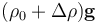, where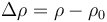represents the density variation with respect to the reference density. This yields: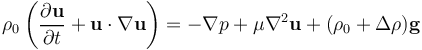To avoid having to evaluate the fluid density based on the local temperature, the buoyancy term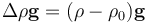can further be rewritten as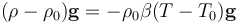, where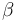is the coefficient of thermal expansion. For ideal gases,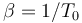and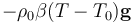becomes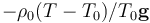. Again, this is only valid when temperature and, therefore, density variations are small: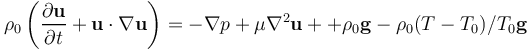### Pressure Shift

The Boussinesq approximation is valid only when \Delta \rho << \rho_{0} . To avoid potential round-off errors in the calculation of the buoyancy term,, the pressure and buoyancy terms on the right-hand side of the Navier-Stokes equations,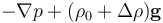, are often rewritten as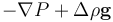, with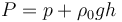, where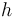represents the elevation.

The equations for the conservation of momentum yield: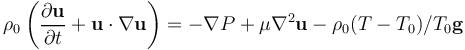The change fromto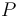is referred to as a pressure shift.

Published: April 2, 2015